# How to Add Fractions with Like Denominators

1. Math Lessons >

### How to Add Like Fractions

Before we get started, let’s brush up on what like fractions are. When two fractions have the same denominators, we call them like fractions. The good news is adding like fractions is as easy as pie!

Let’s take an example. Look at this whole pepperoni pizza cut into 8 equal parts.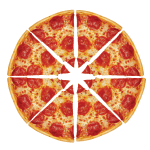If Jake ate 38 and Amanda ate 28 of the pizza, how much pizza did they eat altogether?

Jake ate 3 out of the 8 slices.38

Amanda ate 2 out of the 8 slices.28

The pizza they ate all-together would be:38 + 28 = 58.

So, Jake and Amanda ate 5 out of the 8 slices or 58 of the pizza altogether.

### Adding Fractions Using a Number Line

Let’s take another example and learn to add like fractions using a number line.

Linda walked 49 of a mile to reach the library. She walked 39 of a mile to reach the park. How many miles did Linda walk altogether?

Here, the two fractions refer to the same whole 9. So, we divide the number line into 9 equal parts as shown.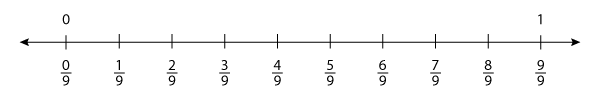Now, let’s draw hops to represent the distance covered by Linda.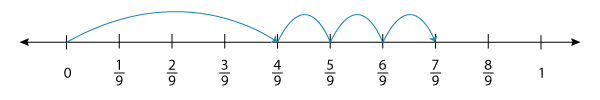The first hop depicts 49 of a mile, and rest of the hops show 39 of a mile. The endpoint 79 denotes the total distance covered or the sum of the two fractions.

Framing a fraction-addition equation for the model, we get:

49 + 39 = 79.

So, Linda walked 79 of a mile in all.

### Adding Like Fractions - Step by Step

Now that we have learnt to add like fractions with the help of real-life examples, let’s explore like-fraction addition presented as math problems!

Recollect these two points whenever you add like fractions.

While adding like fractions, we don’t add the denominators. We simply retain them.

We add only the top numbers and write the sum over the common denominator.

Now, let’s try this: 513 + 713.

Combining the numerators: 5 + 713.

So we have, 513 + 713 = 1213.

### Simplifying the Sum - An Added Step

Here’s an example with an added step. Let’s work it out.

615 + 315

= 6 + 315[Combining the numerators]

And now comes the added step. The fraction 915 can be reduced further. That is to say, it can be simplified to the lowest terms. To reduce it,

Factor the numerator and denominator: 915 = 3 x 33 x 5.

Cancel the common factors if any: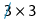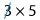.

Thus, 615 + 315 = 35.

Now that we know the steps, let’s implement them to add three fractions.

### How to Add Three Like Fractions

Adding three fractions doesn’t make much of a difference in the steps involved. Let’s look at an example.

Example

Find 734 + 634 + 434.

734 + 634 + 434

[Expression given]

= 7 + 6 + 434

[Combining the numerators]

= 1734

= 172 x 17

[Factoring the denominator]

= 12

[Simplifying]

So, we have 734 + 634 + 434 = 12.

1) Find the sum of the fractions represented by the models.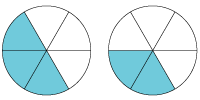The addends represented by the models are 37 and 27.

The sum of the fractions is 37 + 27 = 57.

2) Sarah cut a cake into 12 equal pieces. She gave away 312 of the cake to her friends. She let her family have 412 of it. How much cake did Sarah give away in all?

To friends: 312 of the cake.

To family: 412 of the cake.

The quantity of cake given away in all: 312 + 412 = 712.

3) Our city recorded a rainfall of 15 of an inch in the morning. It registered a rainfall of 25 of an inch at night. Draw a number line to represent the scenario and find the total rainfall.

The number line model that represents the given scenario is: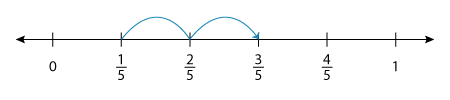The addition equation is 15 + 25 = 35.

So, the total rainfall the city saw is 35 of an inch.

i) 14 + 34 = 1

ii) 36 + 26 = 56

iii) 318 + 518 + 418 = 23

iv) 516 + 216 + 816 = 1516

### The Lesson in Short

• Fractions with the same denominators are called like fractions.

• Combine the numerators.

• Add the numbers on the numerator.

• Simplify or reduce the sum to its lowest terms, if required.Excel in the process by practicing our free printable Adding Like Fractions worksheets.

Progress
Score

Restart Quiz